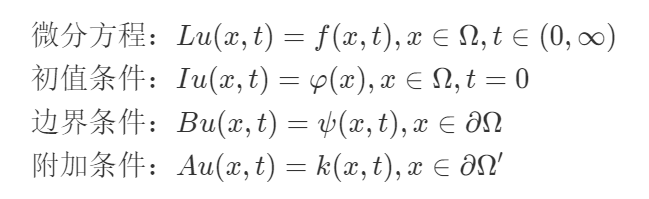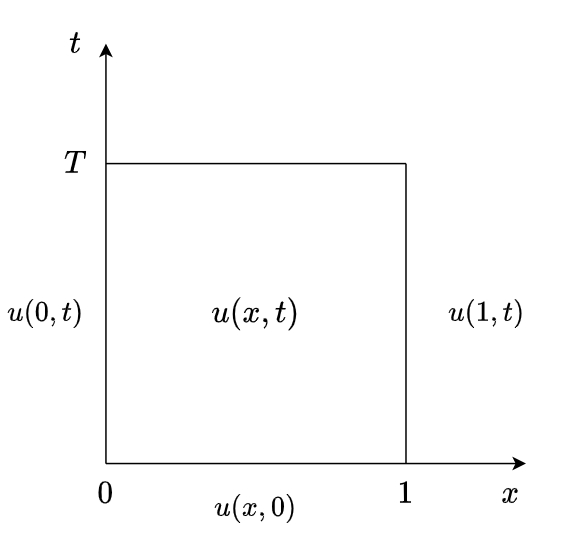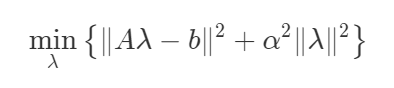# 抱歉，您的浏览器无法访问本站

## 反问题与不适定问题

### 反问题描述

note quote 一对问题称为是互逆的,如果一个问题的构成(已知数据)需要另一个问题解的(部分)信息.把其中一个称为正问题（direct problem）,另一个就称为反问题（inverse problem）.-Joseph B.Keller

### 不适定问题的三个判断标准

• 问题的解是存在的；
• 问题的解是唯一的；
• 问题的解是稳定的；

$$Ax=y, A: X \rightarrow Y 的线性紧算子$$

1、解的存在性：$\forall y \in Y, \exist x \in X,$ 使得 $Ax=y.$
2、解的唯一性：$\forall y_1, y_2 \in Y, y_1 \neq y_2,$ 有 $Ax_1=y_1, Ax_2=y_2,$ 使得 $x_1 \neq x_2.$
3、解的稳定性（即解的连续性）：若有 $Ax_1=y_1, Ax_2=y_2,$ 则当 $y_1 \rightarrow y_2$ 时, 使得 $x_1 \rightarrow x_2.$

note info 当定解条件（初值条件，边界条件）以及方程中的系数有微小变动时，相应的解也只有微小变动. 解的稳定性也称为解关于参数的连续依赖性.

## 微分方程

• 微分方程的定解条件：即初值条件和边界条件；
• 三类边界条件
• 第一类：狄利克雷边界条件（Dirichlet boundary condition）也被称为常微分方程或偏微分方程的“第一类边界条件”，指定微分方程的解在边界处的值. 求出这样的方程的解的问题被称为狄利克雷问题.
• 第二类：诺伊曼边界条件（Neumann boundary condition) 也被称为常微分方程或偏微分方程的“第二类边界条件”. 诺伊曼边界条件指定了微分方程的解在边界处的微分.
• 第三类：Robbin条件/混合边界条件，未知函数在边界上的函数值和外法向导数的线性组合.
• 偏微分方程三大问题
• 初边值问题或混合问题：偏微分方程 + 初值条件 + 边界条件；
• 初值问题或 Cauchy 问题：偏微分方程 + 初值条件；
• 边值问题：偏微分方程 + 边界条件；
• 方程式与方程组
• 方程式：方程个数为 1；
• 方程组：方程个数大于 1；
• 欠定与超定
• 欠定：方程个数少于未知函数个数；
• 超定：方程个数多于未知函数个数；
• 方程（组）中出现的未知函数的最高阶偏导数的阶数称为方程（组）的阶数.

### 数学物理微分方程反问题的分类• 参数识别问题：算子 $L$ 未知（通常 $L$ 的结构是已知的，未知的为算子中的参数）；
• 寻源反问题：右端方程源项 $f(x,t)$ 未知；
• 逆时反问题：$\varphi(x)$ 条件未知时，附加条件为系统某一时刻的状态，该反问题从后面的状态去确定初始状态；
• 边界控制问题：边界条件 $\psi(x,t)$ 未知；
• 几何反问题：区域边界 $\partial \Omega$ 未知；

## 热传导方程

Direct heat conduction problems (DHCPs) 直接热传导问题, Inverse heat conduction problem (IHCPs) 逆热传导问题

### 热传导正/反问题

• 热传导反问题：在热交换情形下，通过研究物体内部或边界的一点或多点温度分布信息来反演热源项、初始条件、边界条件、物理的几何条件等未知量.
• 热传导方程非特征 Cauchy 问题的：典型的热传导方程的非特征Cauchy问题是通过一部分边界上的或者内部的数据来判定另一部分边界的热流.

### 一维热传导方程初边值问题## 处理热传导方程非特征 Cauchy 问题的相关方法

### 基本解方法

$$\begin{array}{c} \tilde{u}(x) = \sum_{i = 1}^{N} a_{i} u^{*}\left(x-\mu_{i}\right) \end{array}$$

### 正则化方法

• Tikhonov 正则化方法• 确定式方法
• 偏差原则
• 拟最优方法
• 启发式方法
• 广义交叉核式
• L-曲线法则

## 参考

•  张智倍. 热传导方程反问题的若干方法研究[D]. 哈尔滨: 哈尔滨工业大学, 2010.
•  臧顺全. 热传导方程正问题和反问题的数值解研究[D]. 西安: 西安理工大学, 2019.
•  王明新. 数学物理方程[M]. 清华大学出版社, 2005: 1-171.
•  贾现正. 热传导方程中的若干反问题[D]. 上海: 复旦大学, 2005.
•  金邦梯. 一类椭圆型偏微分方程反问题的无网格方法[D]. 杭州: 浙江大学, 2005.